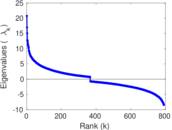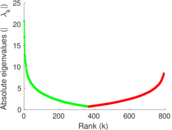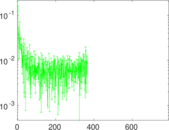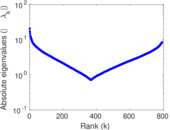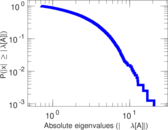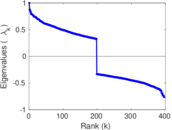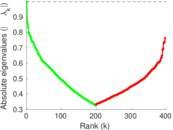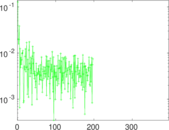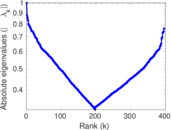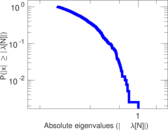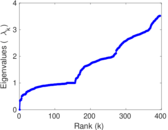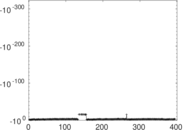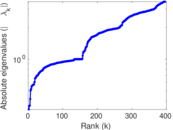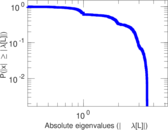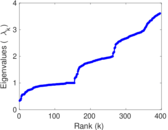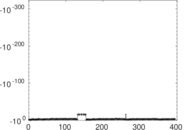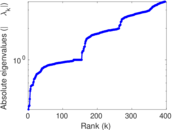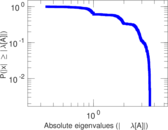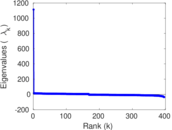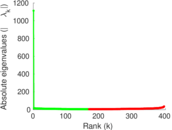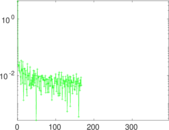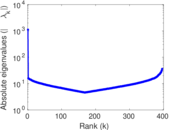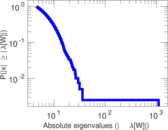U. Rovira i Virgili

This is the email communication network at the University Rovira i Virgili in Tarragona in the south of Catalonia in Spain. Nodes are users and each edge represents that at least one email was sent. The direction of emails and the number of emails between two persons are not stored.

 Code A@ Internal name arenas-email Name U. Rovira i Virgili Data source http://deim.urv.cat/~aarenas/data/welcome.htm AvailabilityDataset is available for download Consistency checkDataset passed all tests Category Communication network Node meaning User Edge meaning Communication Network format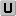Unipartite, undirected Edge typeUnweighted, no multiple edges LoopsDoes not contain loops Orientation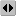Is not directed, but the underlying data is Multiplicity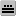Does not have multiple edges, but the underlying data has

Statistics

 Size n = 1,133 Volume m = 5,451 Loop count l = 0 Wedge count s = 96,415 Claw count z = 817,974 Cross count x = 6,550,542 Triangle count t = 5,343 Square count q = 43,591 4-Tour count T4 = 745,290 Maximum degree dmax = 71 Average degree d = 9.622 24 Fill p = 0.008 500 21 Size of LCC N = 1,133 Diameter δ = 8 50-Percentile effective diameter δ0.5 = 3.179 35 90-Percentile effective diameter δ0.9 = 4.475 95 Median distance δM = 4 Mean distance δm = 3.654 76 Gini coefficient G = 0.491 113 Balanced inequality ratio P = 0.314 529 Relative edge distribution entropy Her = 0.942 894 Power law exponent γ = 1.561 09 Tail power law exponent γt = 6.771 00 Tail power law exponent with p γ3 = 6.771 00 p-value p = 0.752 000 Degree assortativity ρ = +0.078 201 0 Degree assortativity p-value pρ = 2.915 87 × 10−16 Clustering coefficient c = 0.166 250 Spectral norm α = 20.747 0 Algebraic connectivity a = 0.332 560 Spectral separation |λ1[A] / λ2[A]| = 1.223 03 Non-bipartivity bA = 0.592 240 Normalized non-bipartivity bN = 0.235 829 Algebraic non-bipartivity χ = 0.334 159 Spectral bipartite frustration bK = 0.008 681 95 Controllability C = 60 Relative controllability Cr = 0.052 956 8

Plots

Fruchterman–Reingold graph drawing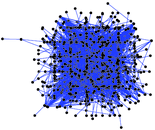Degree distribution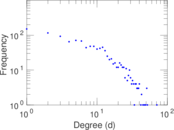Cumulative degree distribution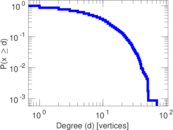Lorenz curve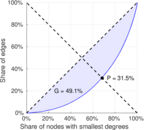Spectral distribution of the adjacency matrix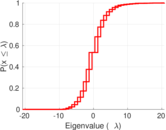Spectral distribution of the normalized adjacency matrix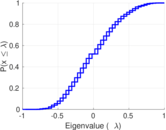Spectral distribution of the Laplacian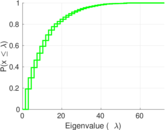Spectral graph drawing based on the adjacency matrix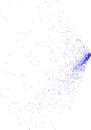Spectral graph drawing based on the Laplacian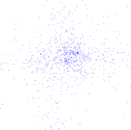Spectral graph drawing based on the normalized adjacency matrix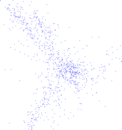Degree assortativity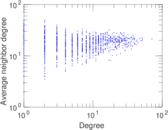Zipf plot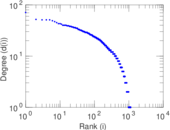Hop distribution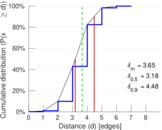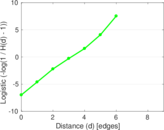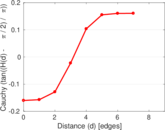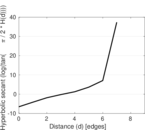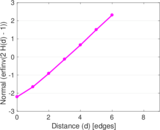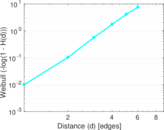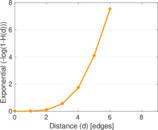Double Laplacian graph drawing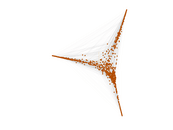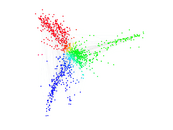Delaunay graph drawing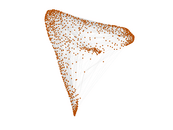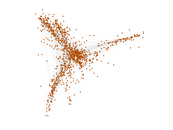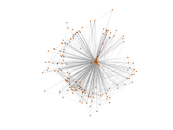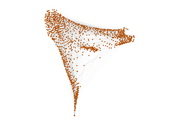Clustering coefficient distribution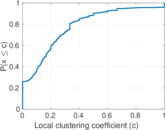Average neighbor degree distribution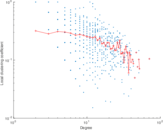SynGraphy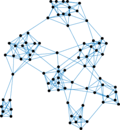Matrix decompositions plots